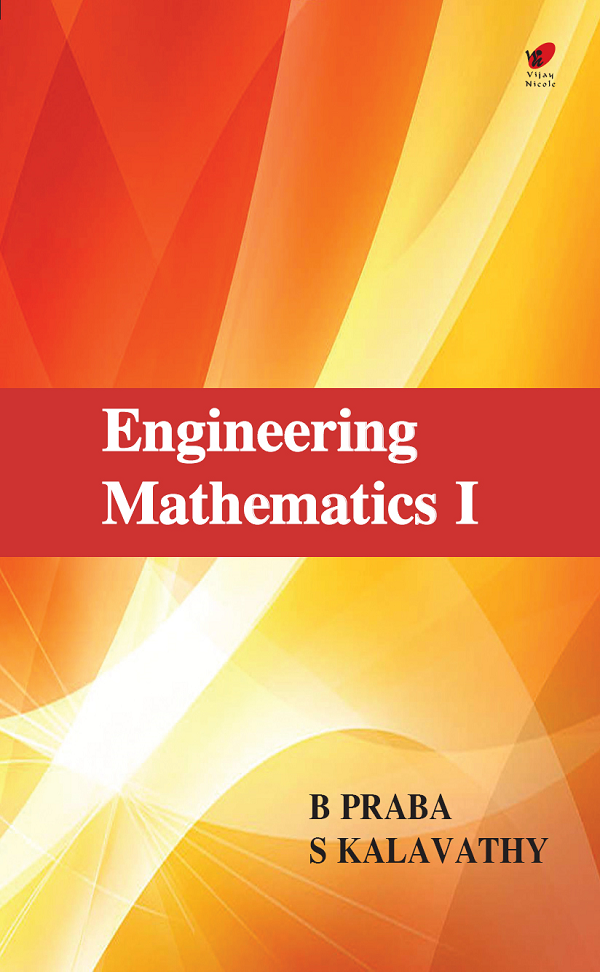Engineering Mathematics I
Subject(s):
ISBN 9789394524095
Publication Date
Pages 438
Description

Designed to cater to the requirements of first year engineering students, this book completely covers the latest syllabus of Anna University Regulation 2017.

Cover
Title Page
Contents
Preface
Unit - I Differential Calculus
Limit, Continuity and Differentiability
Introduction
Limit of a Function
Properties of Limits
Left and Right Limits
Infinite Limits
Continuity
Properties of Continuous Functions
The Derivative
Higher Derivatives
Mean Value Theorem
Rolle’s Theorem
Mean Value Theorem (Lagrange’s)
Alternative Form of Lagrange’s Mean Value Theorem
Cauchy’s Mean Value Theorem
Alternative Form of Cauchy’s Mean Value Theorem
Unit - II Functions of Several Variables
Partial Derivatives
Definition
Total Differentiation
Derivatives of Implicit Functions
Euler’s Theorem for Homogeneous Functions
Total Differential Coefficient
Functions of Functions and Implicit Functions
Taylor’s Theorem
Jacobians
Properties
Maxima and Minima of Functions of Two variables
Definition—Maximum
Definition—Minimum
Working Rule for Finding the Extreme Values of f(x, y)
Constrained Maxima and Minima
Lagrange Method of Multipliers
Unit - III Integral Calculus
Integral Calculus
Definite Integral
Proper Integral
Improper Integral
Definite Integral
Riemann Sum
Riemann Integral
Examples of Riemann Integration From Definition
Definition
(I) Algebraic Formulae
(II) Trigonometry Formulae
Riemann Sum Practice Problems
Fundamental Theorem of Integral Calculus
Integration By Parts
Cancellation of Integrals
Integration By Parts in the Case of Definite Integrals
Integration of Partial Fractions
Rational Function
Partial Fractions
Method of Finding the Constant Quantities
Miscellaneous Solved Examples
Integration of Rational Functions
Definition
Integration of Irrational Algebraic Fractions
Integrations of Rational Functions of x and (ax + b)1/n
Reduction Formulae
Walli’s Formula
Unit - IV Multiple Integrals
Introduction
Double Integrals
Evaluation of Double Integrals
Beta and Gamma Function
Relation between Beta and Gamma Functions
Area as Double Integral
Changing the Order of Integration
Application of Beta Gamma Functions to Multiple Integrals
Transformation from Cartesian Coordinates to Polar Coordinates
Triple Integrals
Evaluation of Triple Integrals
Cartesian Coordinator Triple Integrals
Volume as Triple Integral
Change of Variables
Jacobian
Two Important Results Regarding Jacobians
Change of Variable in the Case of Two Variables
Change of Variables in the Case of Three Variables
Transmission from Cartesian to Polar Coordinates
Transformation from Cartesian to Polar Coordinates
Unit - V Differential Equations
Table of Integration Formulas
Procedural Rules
Basic Formulas
Ordinary Differential Equations
Linear differential Equations with Constant Coefficients
Simultaneous First Order Linear Equations with Constant Coefficient
Linear Equations with Variable Coefficients
Cauchy’s and Legendre’s Linear Equations
The Method of Variation of Parameters
Method of Undetermined Coefficients for Finding the Particular Integral
Solved Question Bank
Index

B Praba is presently Associate Professor, Department of Mathematics, SSN College of Engineering, Chennai. She has 22 years of research and teaching experience and has written books on Discrete Mathematics, Statistics, Random Process and Queuing Theory and Statistics for Management. She has obtained her Ph.D., from Ramanujan Institute for Advanced Study in Mathematics, Chennai.

S Kalavathy is Professor, Department of Mathematics, RMD Engineering College, Chennai. She has over 23 years of experience in teaching Mathematics and has written several books including best sellers on Engineering Mathematics and Operational Research.